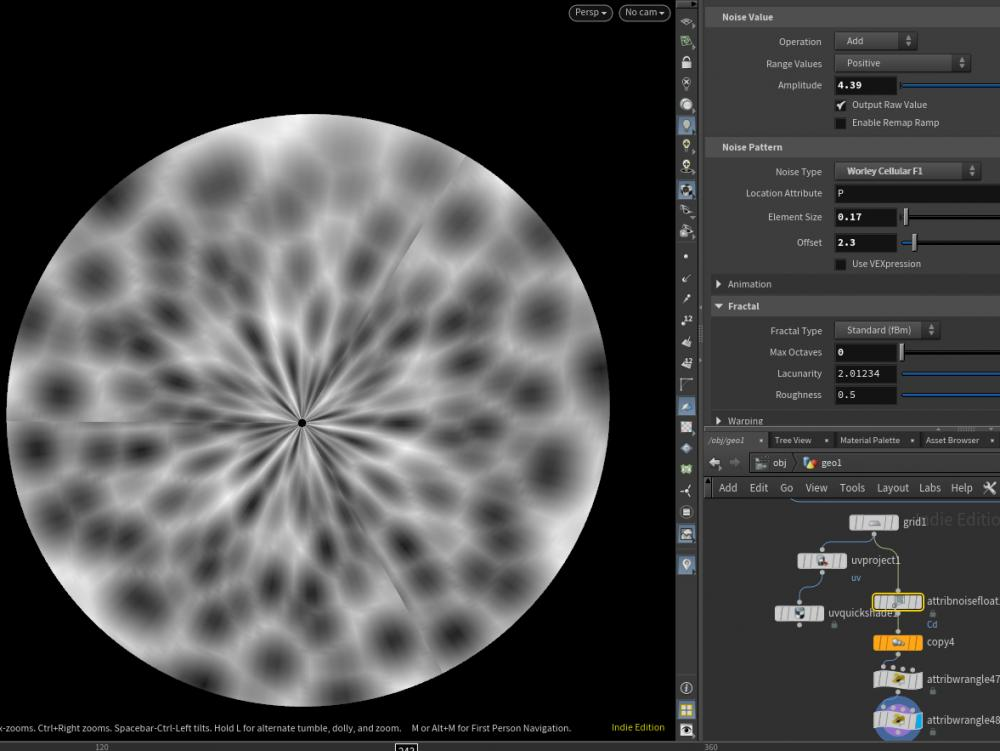## Recommended Posts

Hey guys
I'm trying to reproduce same noise pattern which originally created by blender.

In original video(@00:01:40), author used Mapping node to manipulate UVs.

In houdini, I tried UV Position VOP node but unfortunately can't get reasonable result.

Thanks for any help

Cheers

##### Share on other sites

@akirasun i houdini 2000 ways... BlenderGrid, Noise ,bbox(0, D_XSIZE),Copy Sop, than

```//on prim
vector min, max;
getbbox(min, max);
v@min = min;
v@max = max;```

```vector min = prim(geoself(), "min", 0);
vector max = prim(geoself(), "max", 0);

float height = chf("height");
float rMin = radius;
float rMax = radius + height;
float r = fit(@P.y, min.y, max.y, rMin, rMax);

float radianMin = 0.0;
float radianMax = 2.0 * \$PI;

float x = r * cos(radian);
float y = r * sin(radian);
float z = 0;
@P = set(x, y, z);```•1

##### Share on other sites
8 hours ago, Librarian said:

@akirasun i houdini 2000 ways... BlenderGrid, Noise ,bbox(0, D_XSIZE),Copy Sop, than

```
//on prim
vector min, max;
getbbox(min, max);
v@min = min;
v@max = max;```

```
vector min = prim(geoself(), "min", 0);
vector max = prim(geoself(), "max", 0);

float height = chf("height");
float rMin = radius;
float rMax = radius + height;
float r = fit(@P.y, min.y, max.y, rMin, rMax);

float radianMin = 0.0;
float radianMax = 2.0 * \$PI;

float x = r * cos(radian);
float y = r * sin(radian);
float z = 0;
@P = set(x, y, z);```

Wow, GJ!

## Create an account or sign in to comment

You need to be a member in order to leave a comment

## Create an account

Sign up for a new account in our community. It's easy!

Register a new account